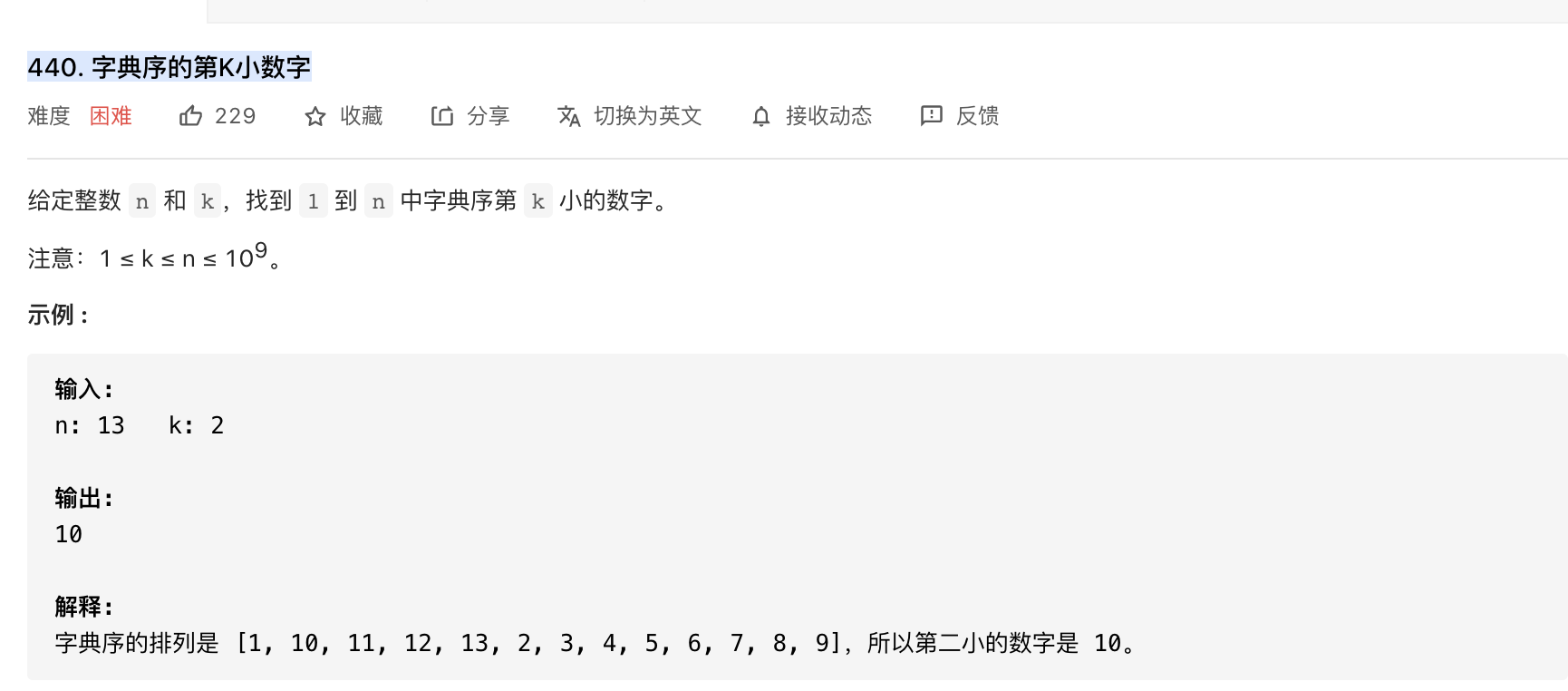【leetcode前500】440. 字典序的第K小数字

2021/8/21 18:15:31 浏览：class Solution:
def findKthNumber(self, n: int, k: int) -> int:
def getCount(prefix,maxn):
cur=prefix
nextn=prefix+1
count=0
while cur<=maxn:
count+=min(maxn+1,nextn)-cur
cur,nextn=cur*10,nextn*10
return count
p=prefix=1
while p<k:
cnt=getCount(prefix,n)
if p+cnt>k:
prefix*=10
p+=1
else:
prefix+=1
p+=cnt
return prefix